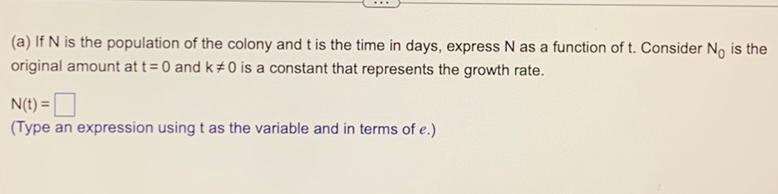Question:

# (a) If N is the population of the colony and t is the time in days, express N as a function of t. Consider N₀ is the original amount at t= 0 and k≠0 is a constant that represents the growth rate. N(t)(a) If N is the population of the colony and t is the time in days, express N as a function of t. Consider N₀ is the original amount at t= 0 and k≠0 is a constant that represents the growth rate. N(t) = □ (Type an expression using t as the variable and in terms of e.)